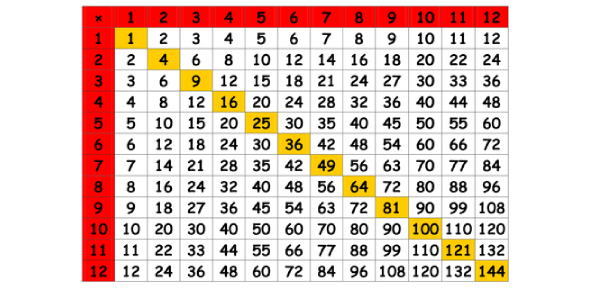# A 12x12 Timed Multiplication Test

50 Questions | Attempts: 35506
ShareSettingsTest your memory of the 12x12 multiplication table with this timed multiplication speed test. Try to be both fast and accurate! At the end of the exam, you receive a grade based on the number of questions you answered correctly. You can also review the questions you missed. Grading Scale: 93%-100% = A 86%-92% = B 78%-85% = C 70%-77% = D Below 70% = F

• 1.
5 x 4 =
• 2.
9 x 9 =
• 3.
12 x 3 =
• 4.
7 x 4 =
• 5.
9 x 6 =
• 6.
8 x 5 =
• 7.
9 x 12 =
• 8.
11 x 5 =
• 9.
2 x 3 =
• 10.
4 x 10 =
• 11.
8 x 9 =
• 12.
12 x 11 =
• 13.
7 x 3 =
• 14.
4 x 3 =
• 15.
8 x 4 =
• 16.
5 x 7 =
• 17.
9 x 4 =
• 18.
12 x 9 =
• 19.
10 x 8 =
• 20.
9 x 7 =
• 21.
6 x 6 =
• 22.
0 x 0 =
• 23.
8 x 7 =
• 24.
5 x 5 =
• 25.
9 x 5 =

## Related TopicsBack to top
×

Wait!
Here's an interesting quiz for you.